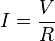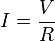# Ohm's Law Homework

## Homework Statement

1. A nine volt battery supplies power to a cordless curling iron with a resistance of 18 ohms. How much current is flowing through the curling iron?
2. A 110 volt wall outlet supplies power to a strobe light with a resistance of 2200 ohms. How much current is flowing through the strobe light?
3. A CD player with a resistance of 40 ohms has a current of 0.1 amps flowing through it. Calculate how many volts supply the CD player?
4. A small electrical pump is labeled with a rating of 3 amps and a resistance of 40 ohms. What voltage was it meant to run at?
5. A lamp is plugged into the wall outlet, which is providing 110 volts. An ammeter attached to the lamp shows 2 amps flowing through the circuit. How many ohms of resistance is the lamp providing?

## The Attempt at a Solution

Don't know where to start.

kuruman
Homework Helper
Gold Member

As you stated in the title, Ohm's Law. Simply plug what you know into Ohm's Law and solve for the unknown value.

As you stated in the title, Ohm's Law. Simply plug what you know into Ohm's Law and solve for the unknown value.

I'm lot though I know about it but they tell you what Ohms law is and it confuses me.kuruman
Homework Helper
Gold Member
I'm lot though I know about it but they tell you what Ohms law is and it confuses me.What confuses you? Can you state in plain English and without equations what Ohm's Law says?

What do I use to figure out the problem?
The only thing it gives me is 18ohms for the first one.

Just completely lost and when I try to read about the Ohms law none of it makes sense.

kuruman
Homework Helper
Gold Member
Does V = I R make more sense? Some times Ohm's Law is written in this form.

it is very simple..

the current equals the voltage divided by the resistance...
and in first problem, the voltage equals 9 V (9V battery) and the resistance is 18 ohms....

so try to figure it out...

So the current is 2? What is that measured in, AMPS?

kuruman
Homework Helper
Gold Member
So the current is 2? What is that measured in, AMPS?
Look it up.

no... voltage divided by the resistance = 9 / 18 = 1/2....

and yes, current is measured in amps

1. Since V and R are known, solve for I which is current by dividing both sides of the equation by R.

V = I x R
R R

I = V
R

I = 9
18

I = 0.5 Amps

2. Current = 0.05 Amps

I managed to get the first 2 done.

No I'm stuck on the last 3.

uhh...it doesn't seem like you are even trying.. doesn't physics require you to have some knowledge of algebra at least...?

you already have the equation right there: V = I x R
all the problems give you 2 of the unknown variables.. why can't you solve for the one remaining variable?